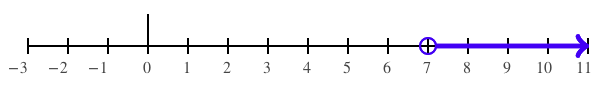# RBSE Maths Class 11 Chapter 6: Important Questions and Solutions

RBSE Solutions for Class 11 Maths Chapter 6 – Linear inequalities are available here. The important questions and solutions of Chapter 6, available at BYJU’S, contain step by step explanations along with the solutions. All these important questions are based on the new pattern prescribed by the RBSE. Students can also get the syllabus and textbooks on RBSE Class 11 solutions.

Chapter 6 of the RBSE Class 11 Maths will help the students to solve problems related to inequalities, algebraic solutions of linear inequalities, graphical solution of linear inequalities, solution of the system of linear inequalities. After referring to these important questions and solutions, you can refer to 2020-2021 solutions for the chapter here.

### RBSE Maths Chapter 6: Textbook Important Questions and Solutions

Question 1: Solve the following inequalities.

[i] 4x + 4 < 5x + 7

[ii] 3x – 9 > 5x – 1

Solution:

[i] 4x + 4 < 5x + 7

Subtracting 7 from both the sides, we get

4x + 4 – 7 < 5x + 7 – 7

4x – 3 < 5x

Subtracting 4x from both the sides,

4x – 3 – 4x < 5x – 4x

x > – 3

The solutions of the inequality given are defined by the real numbers which are greater than -3.

The required solution set is (-3, ∞)

[ii] 3x – 9 > 5x – 1

3x – 9 > 5x – 1

Adding 9 to both the sides, we get

3x – 9 + 9 > 5x – 1 + 9

3x > 5x + 8

Subtracting 5x from both the sides,

3x – 5x > 5x + 8 – 5x

-2x > 8

Divide both sides by -2

-2x / -2 < 8 / -2

x < -4

The solutions of the given inequality are defined by all the real numbers which are less than -4.

The required solution set is (-∞, -4).

Question 2: Solve the given inequality for the real value of x: x + x / 2 + x / 4 < 12.

Solution:

x + x / 2 + x / 4 < 12

Taking as common from the terms we get,

x [1 + (1 / 2) + (1 / 4)] < 12

On taking the LCM,

x {[4 + 2 + 1] / 4} < 12

7x / 4 < 12

Dividing by 12 on both sides

[7x / 4 * 12] < [12 / 12] [7x / 48] < 1

7x < 48

Divide by 7 on both sides

[7x / 7] < [48 / 7]

x < [48 / 7]

Question 3: Solve the inequality 3 (x – 2) / 6 ≤ 5 (2 – x) / 4.

Solution:

3 (x – 2) / 6 ≤ 5 (2 – x) / 4

Apply the fraction cross multiplication,

3 (x – 2) * 4 ≤ 6 * 5 (2 – x)

12 (x – 2) ≤ 30 (2 – x)

12x – 24 ≤ 60 – 30x

12x – 24 + 24 ≤ 60 – 30x + 24

12x ≤ 84 – 30x

12x + 30x ≤ 84 – 30x + 30x

42x ≤ 84

Divide both sides by 42

x ≤ 2

Question 4: Graph the following inequalities.

[i] 2x + y < 3

[ii] y < x2

[iii] y < 5x + 1

[iv] 4y + 11 < 9

[v] x < y6

Solution:

[i] 2x + y < 3

x < [-1 / 2] y + [3 / 2]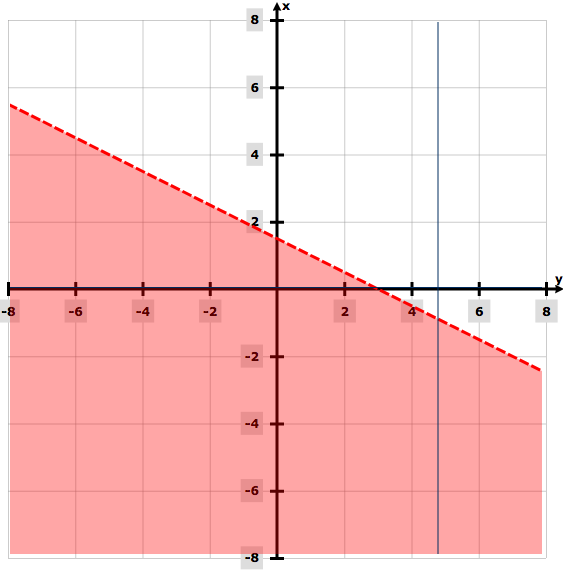[ii] y < x2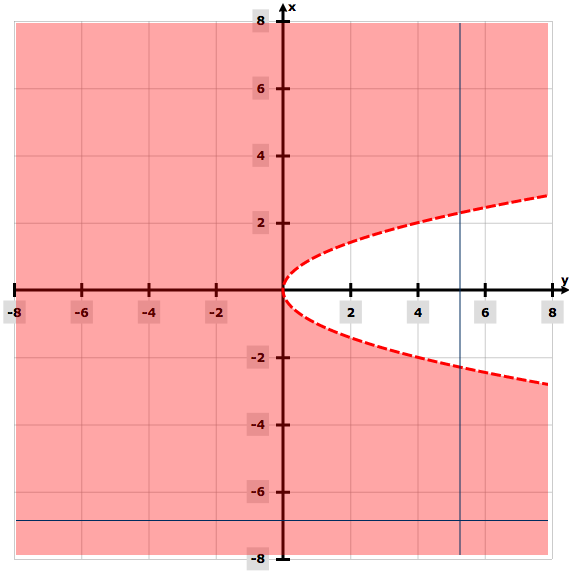[iii] y < 5x + 1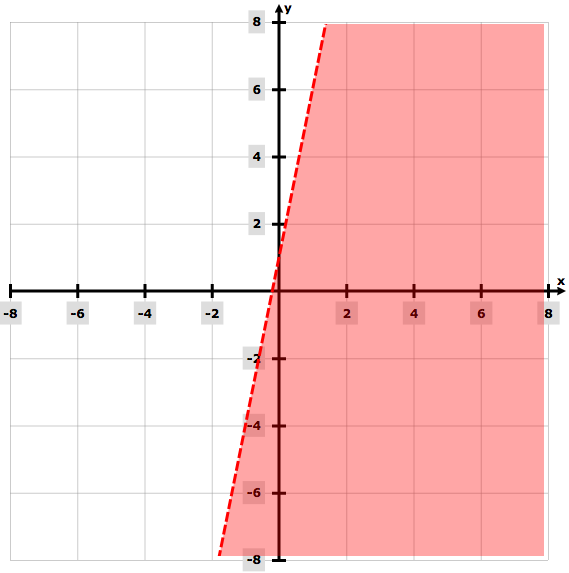[iv] 4y + 11 < 9

(4y + 11) + (-9) < 9 + (-9)

4y + 11 – 9 < 9 – 9

4y + 2 < 0

y < (-1 / 2)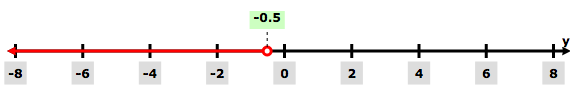[v] x < y6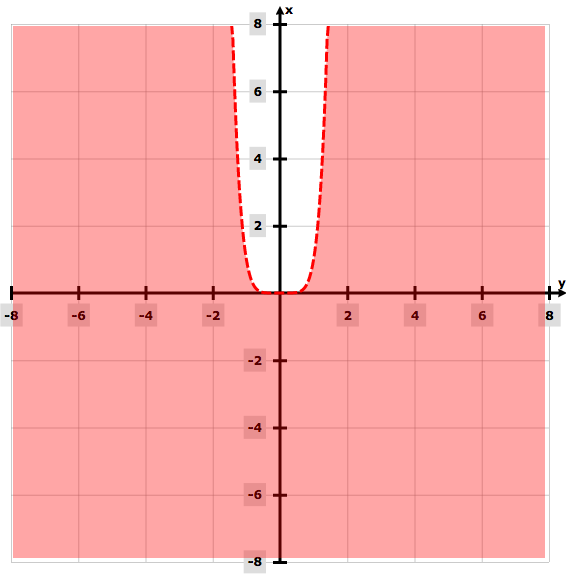Question 5: Solve the following system of inequalities.

[i] 4x + y ≥ 3 , x + 8y ≤ 16

[ii] 3x + y ≥ 6, 9x – y < 9

[iii] 3x – y > 1, x – 4y < – 1

[iv] 4x – y > 1, x – 3y < – 1

Solution:

[i] 4x + y ≥ 3 , x + 8y ≤ 16

4x + y ≥ 3

Subtract 4x on both sides

4x + y – 4x ≥ 3 – 4x

y ≥ 3 – 4x

x + 8y ≤ 16

Subtract x on both sides

x + 8y – x ≤ 16 – x

8y ≤ 16 – x

Divide both sides by 8

[8y / 8] ≤ [16 – x] / 

y ≤ [16 – x] / 

The solution is unbounded.

[ii] 3x + y ≥ 6, 9x – y < 9

3x + y ≥ 6

Subtract 3x on both sides

3x + y – 3x ≥ 6 – 3x

y ≥ 6 – 3x

9x – y < 9

Subtract 9x on both sides

9x – y – 9x < 9 – 9x

– y < 9 – 9x

Multiply both sides by (-1) to reverse the inequality

y > – 9 + 9x

The solution is unbounded.

[iii] 3x – y > 1, x – 4y < – 1

3x – y > 1

Subtract 3x on both sides

3x – y – 3x > 1 – 3x

– y > 1 – 3x

Multiply both sides by (-1) to reverse the inequality

y < – 1 + 3x

x – 4y < – 1

Subtract x on both sides

x – 4y – x < – 1 – x

– 4y < – 1 – x

Multiply both sides by (-1) to reverse the inequality

4y > (1 + x)

Divide both sides by 4

y > [1 + x] / 

The solution is unbounded.

[iv] 4x – y > 1, x – 3y < – 1

4x – y > 1

Subtract 4x on both sides

4x – y – 4x > 1 – 4x

– y > 1 – 4x

Multiply both sides by (-1) to reverse the inequality

y < -1 + 4x

x – 3y < – 1

Subtract x on both sides

x – 3y – x < – 1 – x

– 3y < – 1 – x

Multiply both sides by (-1) to reverse the inequality

3y > 1 + x

The solution is unbounded.

Question 6: Solve the following inequalities using a number line.

[i] 4x + 3 ≤ 23

[ii] 5x + 9 < 8

[iii] 5x – 1 > 26

[iv] 3 (x + 7) > 2 – x

[v] 6 – x > 13 – 2x

Solution:

[i] 4x + 3 ≤ 23

Subtract 3 from both sides.

4x + 3 − 3 ≤ 23 − 3

4x ≤ 20

Divide both sides by

4x / 4 ≤ 20 / 4

x ≤ 5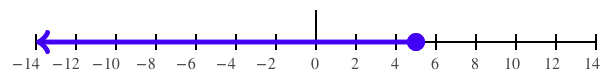[ii] 5x + 9 < 8

Subtract 9 from both sides.

5x + 9 − 9 < 8 − 9

5x < −1

Divide both sides by 5.

5x / 5 < −1 / 5

x < −1 / 5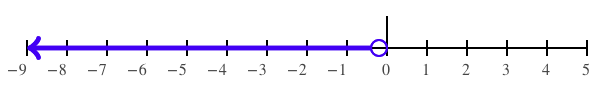[iii] 5x – 1 > 26

5x − 1 + 1 > 26 + 1

5x > 27

Divide both sides by 5.

5x / 5 > 27 / 5

x > 27 / 5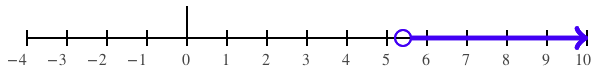[iv] 3 (x + 7) > 2 – x

⇒ 3x + 21 > 2 – x

⇒ 3x + x > 2 – 21

⇒ 4x > -19

⇒ x > -19 / 4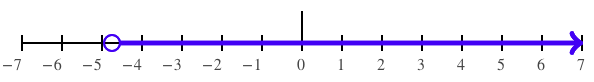[v] 6 – x > 13 – 2x

6 – 13 > – 2x + x

-7 > -x

Multiply both sides by (-1) to reverse the inequality

x > 7